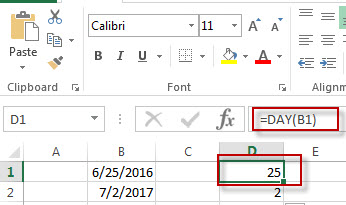# Excel Day Function

This post will guide you how to use Excel DAY function with syntax and examples in Microsoft excel.

### Description

The Excel DAY function returns a day of a date (from 1 to 31)

The DAY function is a build-in function in Microsoft Excel and it is categorized as a DATE and TIME Function.

The DAY function is available in Excel 2016, Excel 2013, Excel 2010, Excel 2007, Excel 2003, Excel XP, Excel 2000, Excel 2011 for Mac.

### Syntax

The syntax of the DAY function is as below:

`= DAY (date_value)`

### Example

The below examples will show you how to use Excel DAY Function to return the day of a date.

#1 =DAY(B2)Note:  The above excel formula will return the day of the date in Cell B1.

### Related Functions

• Excel YEAR function
The Excel YEAR function returns a four-digit year from a given date value, the year is returned as an integer ranging from 1900 to 9999. The syntax of the YEAR function is as below:=YEAR (serial_number)…
• Excel MONTH function
The Excel MONTH function returns the month of a date represented by a serial number. And the month is an integer number from 1 to 12. The syntax of the MONTH function is as below:=MONTH (serial_number)…
• Excel DATEVALUE Function
The Excel DATEVALUE function returns the serial number of date. And it can be used to convert a date represented as text format into a serial number that recognizes as a date format.The syntax of the DATEVALUE function is as below:=DATEVALUE(date_text)…
• Excel WEEKDAY function
The Excel WEEKDAY function returns a integer value representing the day fo the week for a given Excel date and the value is range from 1 to 7.The syntax of the WEEKDAY function is as below:=WEEKDAY (serial_number,[return_type])…